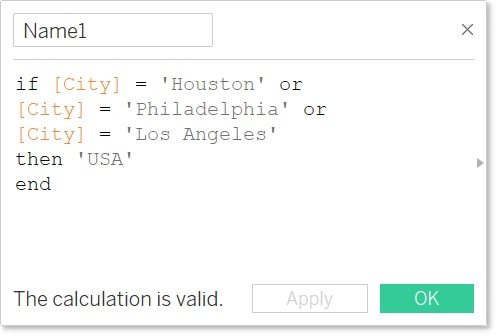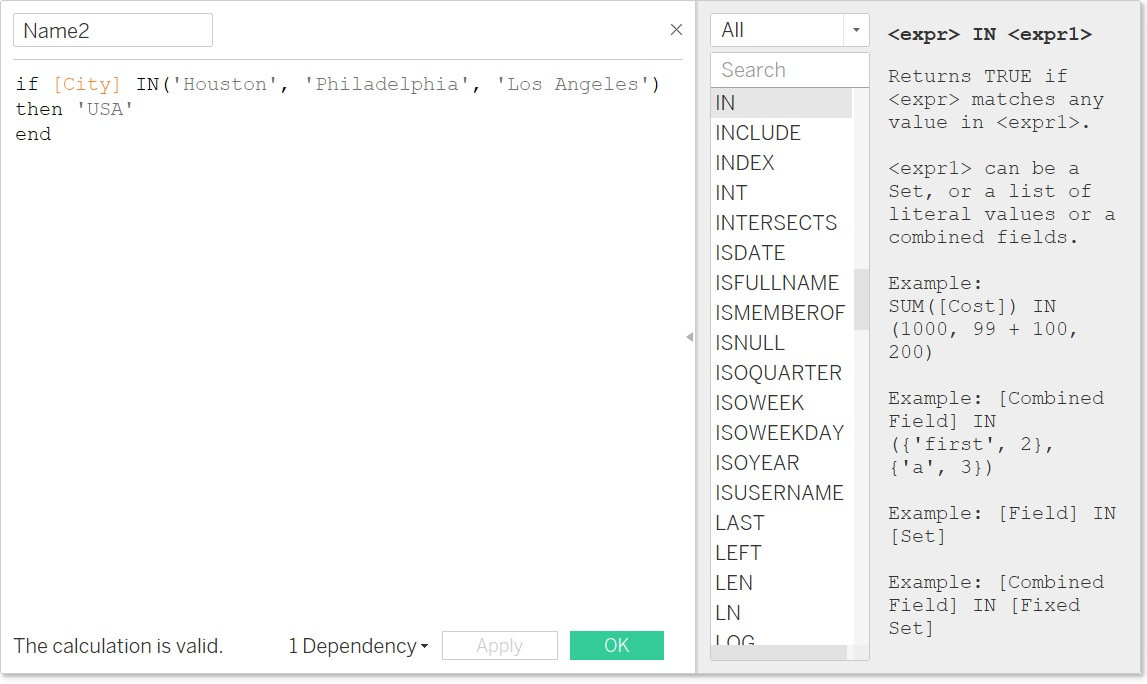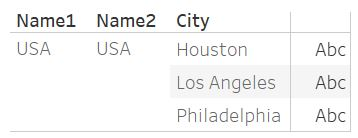(和文はこちらから)

## Introduction:

When using calculated fields in Tableau, it is possible to use the “IN” operator. Its behavior is similar to its SQL equivalent operation. This can bypass multiple “OR” statements by clubbing various clauses together.

The “IN” operator can be used in calculated fields to test whether a specified value matches any value in a list of comma separated values, or a set, or combined fields like City/State combinations etc. The “IN” operator supports string, numeric, date, and boolean data types.

## How to use "IN" operator:

Let us consider a string calculation as shown below:Until now, the “IF” statement would get bigger with multiple “OR” clauses. However, by using the “IN” operator, the same calculation can be simplified as shown below.In both cases the output would be the same as shown below.The “IN” operator in Tableau behaves similarly to its SQL equivalent however, while SQL users can further use the “NOT IN” syntax, the latter is not yet allowed in Tableau.

## Summary:

Use the “IN” operator in various calculated fields and tidy up your work.